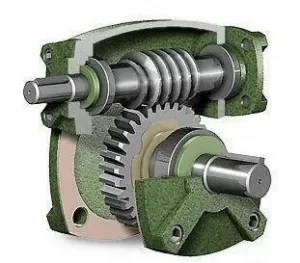What is the transmission efficiency of the worm gear reducer? (worm drive gearbox, worm gearbox calculation)

Worm gearbox efficiency is usually decided by gear ratio and different worm gears design, In the worm gear transmission system, due to the internal gear transmission, part of the energy will be converted into heat energy and gradually disappear.
Efficiency = output shaft HP/input shaft HP x 100%, The efficiency of the worm gear reducer (reducer) depends on the advance angle of the worm, the advance speed, lubricating oil, bearing friction, and material friction coefficient.The reason effect on gear efficiency reason has many factors, the most important factor is the total efficiency of the worm transmission = the transmission efficiency of the worm screw pair × the efficiency of the bearing supporting the worm gear × the transmission efficiency of the splash lubrication.
Transmission efficiency of worm screw pair = tg (lead angle)/tg (lead angle + equivalent friction angle) tg (lead angle) = (number of screw heads of worm × modulus) / worm index circle diameter

It can be seen from the above three formulas that when the worm index circle diameter ↑, tg (lead angle) ↓, lead angle ↓, tg (lead angle + equivalent friction angle) ↓, but due to the existence of the equivalent friction angle, tg (lead angle) decreases faster than tg (lead angle + equivalent friction angle), then the transmission efficiency of the worm screw pair is ↓, and the final total efficiency of the worm transmission ↓

please check the general worm gear efficiency chart

 Worm Gear Ratio Efficiency 10:1 82~85% 20:1 78~82% 30:1 73~76% 40:1 69~75% 50:1 67~72% 60:1 65~70%

Therefore, the index circle diameter of the worm is closely related to the total efficiency of the worm drive.

please contact SDT Transmission™ if you need a worm gear efficiency formula or worm gear efficiency table.